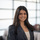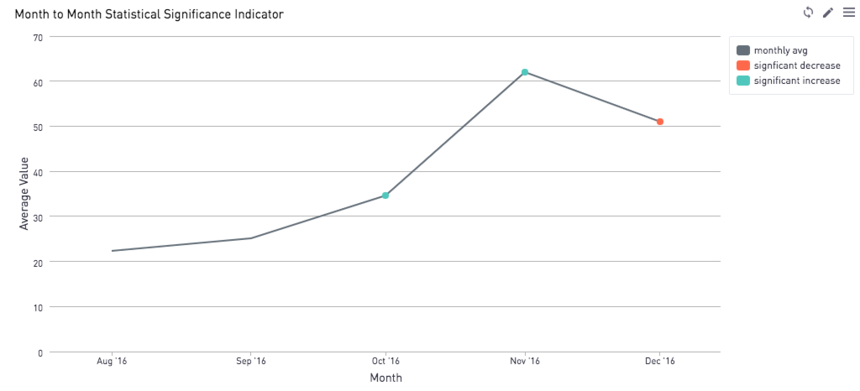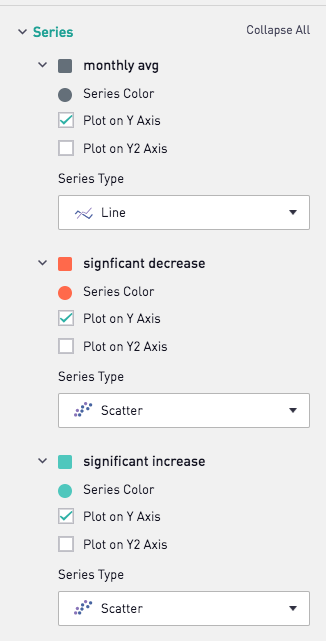# Visualizing Statistical Significance in Samples over Time - RLet's say you're tracking a KPI, and want to see if any changes in your KPI are statistically significant. It would be nice to mark these points on your line graph to call attention to these particular increases and decreases. In the above visualization, we used Periscope's R Integration to calculate statistical significance month over month on the average amount paid per user on a fictional gaming platform.

Our SQL output has 3 columns:

• The user_id
• The month of the observation
• The amount paid per user in that month

We use the R code below to add a column for statistical significance, and also do some other minor transformations on the data frame to prep it for plotting via the Periscope visualization settings. Note that the default p-value threshold is 0.05, but this can be tailored in the final function call at the end of the code block below. In the code below, we override the default 0.05 in favor of a more stringent p-value of 0.01.

Note that the code below assumes a t-distribution and applies a two-tailed test.

```# SQL output is imported as a dataframe variable called "df"
# Use Periscope to visualize a dataframe or show text by passing data to periscope.table() or periscope.text() respectively. Show an image by calling periscope.image() after your plot.

library(dplyr)

summary_metrics <- function(df){
df <- df[,c(2,3)]
df <- df %>%
group_by(my_month) %>%
summarise_all(funs(mean, sd, n()))
return(df)
}

calc_sig <- function(d,i,threshold)
{
x2 <- d\$mean[i+1]
x1 <- d\$mean[i]
sd2 <- d\$sd[i+1]
sd1 <- d\$sd[i]
n2 <- d\$n[i+1]
n1 <- d\$n[i]
t <- (x2 - x1) / sqrt((sd1^2)/n1 + (sd2^2)/n2)
if (x2 > x1)
{
p <- pt(t,df=n1 + n2 - 2, lower=FALSE)
if(p<=threshold/2)
{
return('significant increase')
}
else
{
return('monthly avg')
}
}
else
{
p <- pt(t,df=n1 + n2 - 2, lower=TRUE)
if(p <= threshold/2){
return('signficant decrease')
}
else
{
return('monthly avg')
}
}
}

stat_sig <- function(df, p=0.05){
df= summary_metrics(df)
sig <- c('monthly avg',sapply(1:(nrow(df)-1), calc_sig, d=df, threshold=p))
sig <- data.frame(Reduce(rbind, list(sig)))
colnames(sig) <- c('significance')
df\$sig <- sig\$significance

df2 <- filter(df, sig != 'monthly avg')
df2\$sig = 'monthly avg'
df <- rbind(df,df2)
}

periscope.table(stat_sig(df, p=0.01))```

Finally we apply the following visualization settings. We apply red dots where there is a significant decrease in the KPI from the previous month to the next month, and a green dot if there is a significant increase.Any other methods you like to use for showing significance?

Prefer Python? Check out the Python equivalent of this post!